#Algebra 1 Worksheets

## Monomials and Polynomials Worksheets

Here is a graphic preview for all of the Monomials and Polynomials Worksheets. You can select different variables to customize these Monomials and Polynomials Worksheets for your needs. The Monomials and Polynomials Worksheets are randomly created and will never repeat so you have an endless supply of quality Monomials and Polynomials Worksheets to use in the classroom or at home. Our Monomials and Polynomials Worksheets are free to download, easy to use, and very flexible.

Click here for a Detailed Description of all the Monomials and Polynomials Worksheets.

## Quick Link for All Monomials and Polynomials Worksheets

Click the image to be taken to that Monomials and Polynomials Worksheets.

##### Identifying the Type ofMonomials and PolynomialsWorksheets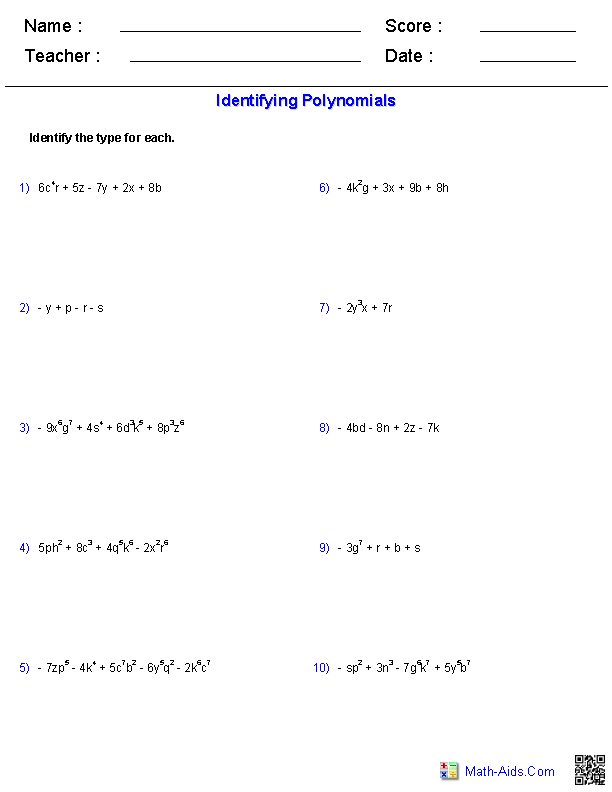##### Identifying the Degree ofMonomials and PolynomialsWorksheets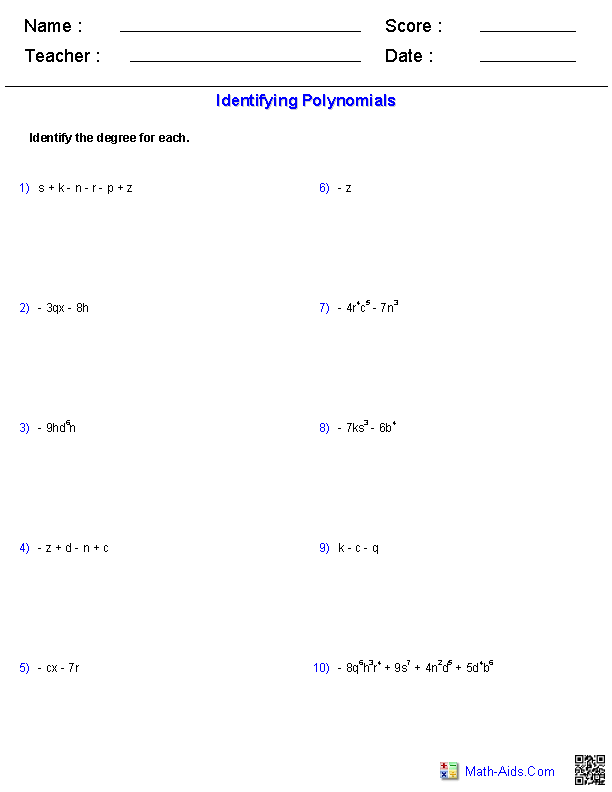##### Naming ofMonomials and PolynomialsWorksheets##### Adding and SubtractingMonomials and PolynomialsWorksheets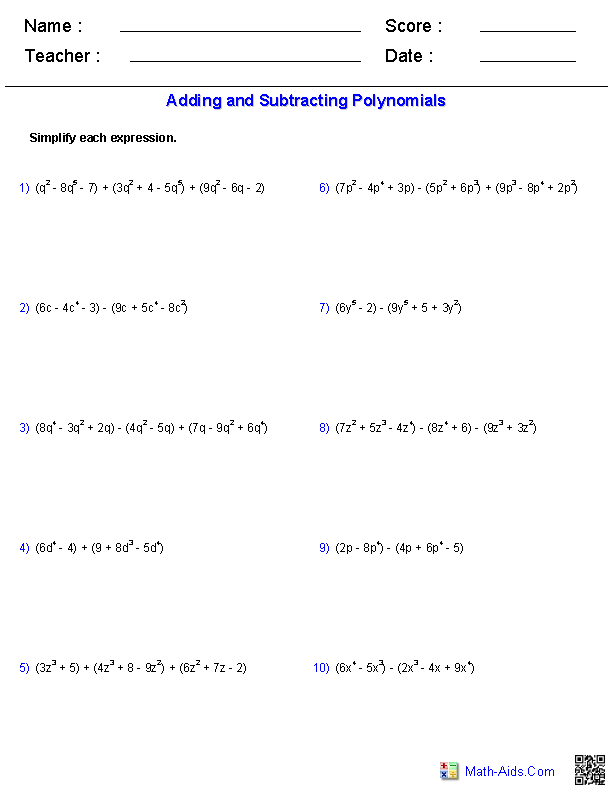##### Multiplying PolynomialsMonomials and PolynomialsWorksheets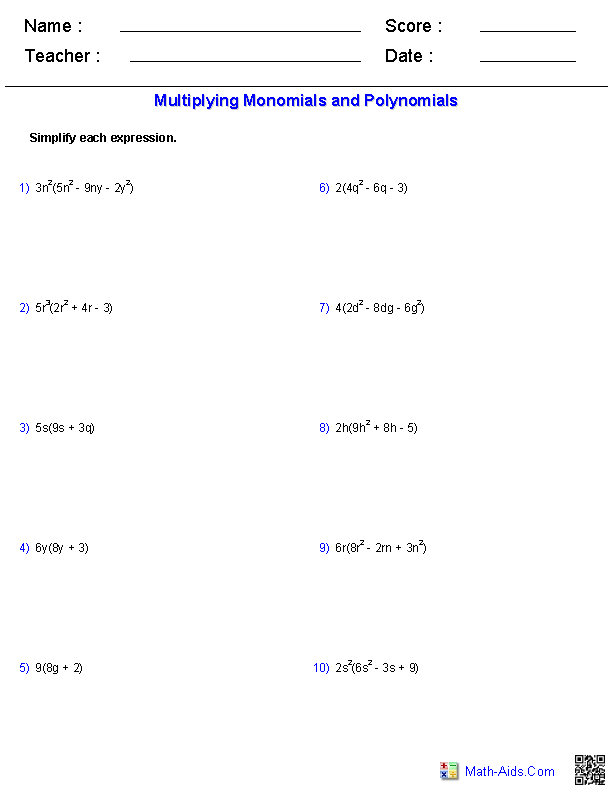##### Multiplying Special CasePolynomials Worksheets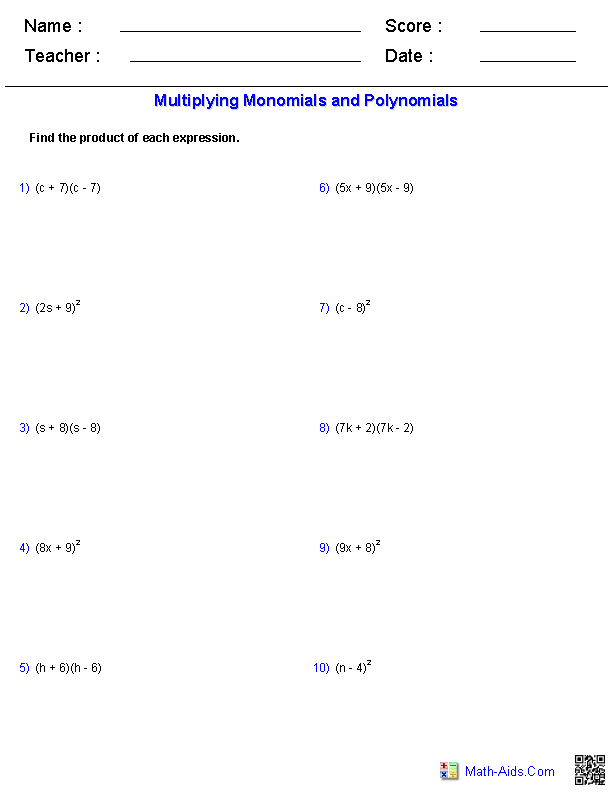##### DividingPolynomials Worksheets##### Dividing Polynomials withLong Division Worksheets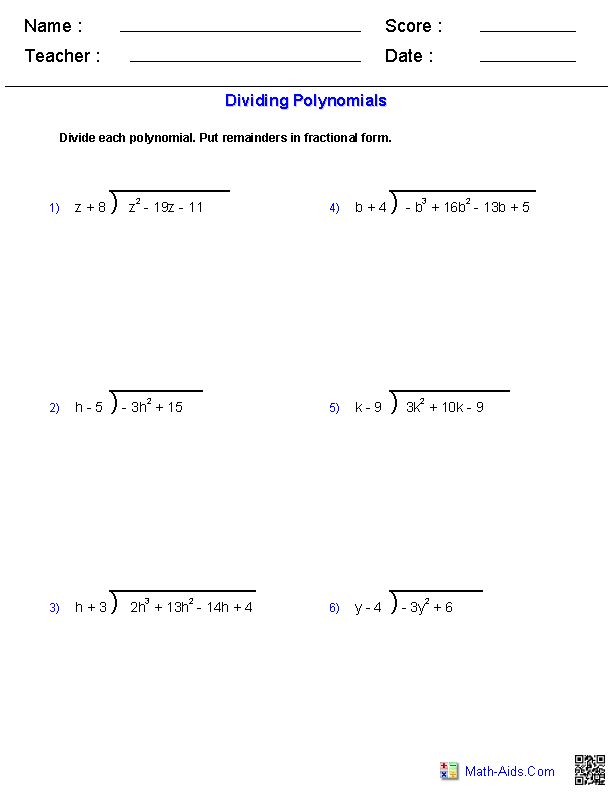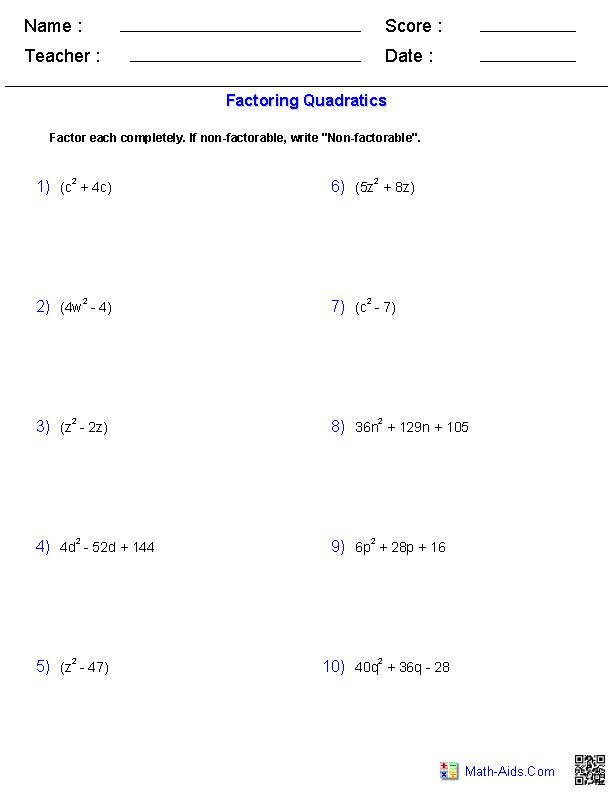##### Factoring Special CasePolynomials Worksheets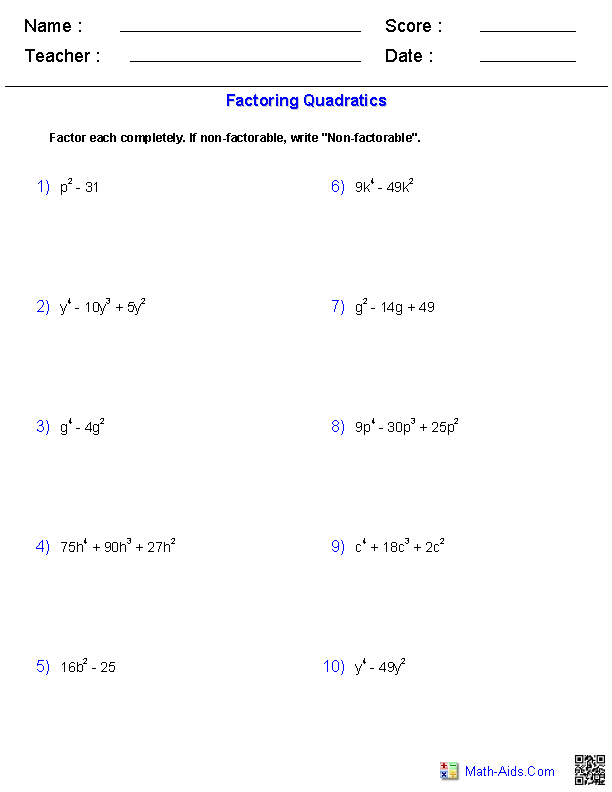##### Factoring by GroupingPolynomials Worksheets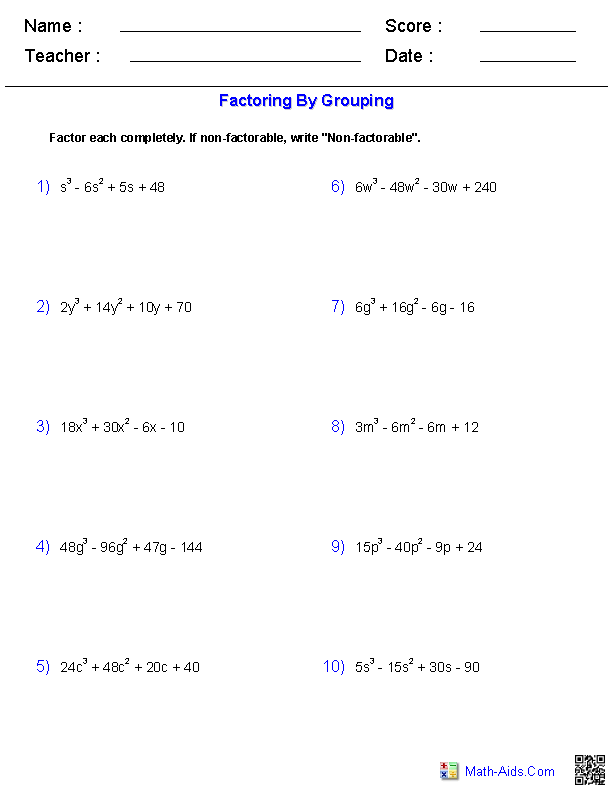Recommended Videos

## Description For All Monomials and Polynomials Worksheets

Identifying the Type of Monomials and Polynomials Worksheets
This monomial and polynomial worksheet will produce problems for identifying the type of monomials and polynomials equations. You may select which type of monomials and polynomials equations to use. This monomial and polynomial worksheet will produce ten problems per page. This monomials worksheet is a good resource for students in the 5th Grade, 6th Grade, 7th Grade, and 8th Grade.

Identifying the Degree of Monomials and Polynomials Worksheets
This monomial and polynomial worksheet will produce problems for identifying the degree of monomials and polynomials equations. You may select which type of monomials and polynomials problem to use. This monomial and polynomial worksheet will produce ten problems per page. This monomials worksheet is a good resource for students in the 5th Grade, 6th Grade, 7th Grade, and 8th Grade.

Naming of Monomials and Polynomials Worksheets
This monomial and polynomial worksheet will produce problems for naming the type and degree of monomials and polynomials equations. You may select which type of monomials and polynomials problem to use. This monomial and polynomial worksheet will produce ten problems per page. This monomials worksheet is a good resource for students in the 5th Grade, 6th Grade, 7th Grade, and 8th Grade.

This polynomial worksheet will produce problems for adding and subtracting polynomials. You may select which type of polynomials problem to use and the range of numbers to use as the constants. This polynomial worksheet will produce ten problems per page. This monomial worksheet will produce ten problems per page. This monomials worksheet is a good resource for students in the 5th Grade, 6th Grade, 7th Grade, and 8th Grade.

Multiplying a Monomial and a Polynomial Worksheets
This monomial and polynomial worksheet will produce problems for multiplying monomials and polynomials. You may select which type of monomials and polynomials problem to use. This monomial and polynomial worksheet will produce ten problems per page. This monomial and polynomial worksheet will produce ten problems per page. This monomials worksheet is a good resource for students in the 5th Grade, 6th Grade, 7th Grade, and 8th Grade.

Multiplying Special Case Polynomial Worksheets
This monomial and polynomial worksheet will produce problems for multiplying special case polynomials. You may select which type of monomials and polynomials problem to use. This monomial and polynomial worksheet will produce ten problems per page. This monomial and polynomial worksheet will produce ten problems per page. This monomials worksheet is a good resource for students in the 5th Grade, 6th Grade, 7th Grade, and 8th Grade.

Dividing Polynomial Worksheets
This monomial and polynomial worksheet will produce problems for dividing polynomials. You may select which type of monomials and polynomials problem to use. This monomial and polynomial worksheet will produce ten problems per page. This monomial and polynomial worksheet will produce ten problems per page. This monomials worksheet is a good resource for students in the 5th Grade, 6th Grade, 7th Grade, and 8th Grade.

Dividing Polynomial with Long Division Worksheets
This monomial and polynomial worksheet will produce problems for dividing polynomials with the problems laid out in the long division format. You may select which type of monomials and polynomials problem to use. This monomial and polynomial worksheet will produce six problems per page. This monomial and polynomial worksheet will produce ten problems per page. This monomials worksheet is a good resource for students in the 5th Grade, 6th Grade, 7th Grade, and 8th Grade.

This monomial and polynomial worksheet will produce problems for factoring quadratic polynomials. You may select which type of monomials and polynomials problem to use. This monomial and polynomial worksheet will produce ten problems per page. This monomial and polynomial worksheet will produce ten problems per page. This monomials worksheet is a good resource for students in the 5th Grade, 6th Grade, 7th Grade, and 8th Grade.

Factoring Special Case Polynomial Worksheets
This monomial and polynomial worksheet will produce problems for factoring special case polynomials. You may select which type of monomials and polynomials problem to use. This monomial and polynomial worksheet will produce ten problems per page. This monomial and polynomial worksheet will produce ten problems per page. This monomials worksheet is a good resource for students in the 5th Grade, 6th Grade, 7th Grade, and 8th Grade.

Factoring by Grouping Polynomial Worksheets
This monomial and polynomial worksheet will produce problems for factoring by grouping. You may select which type of monomials and polynomials problem to use. This monomial and polynomial worksheet will produce ten problems per page. This monomial and polynomial worksheet will produce ten problems per page. This monomials worksheet is a good resource for students in the 5th Grade, 6th Grade, 7th Grade, and 8th Grade.